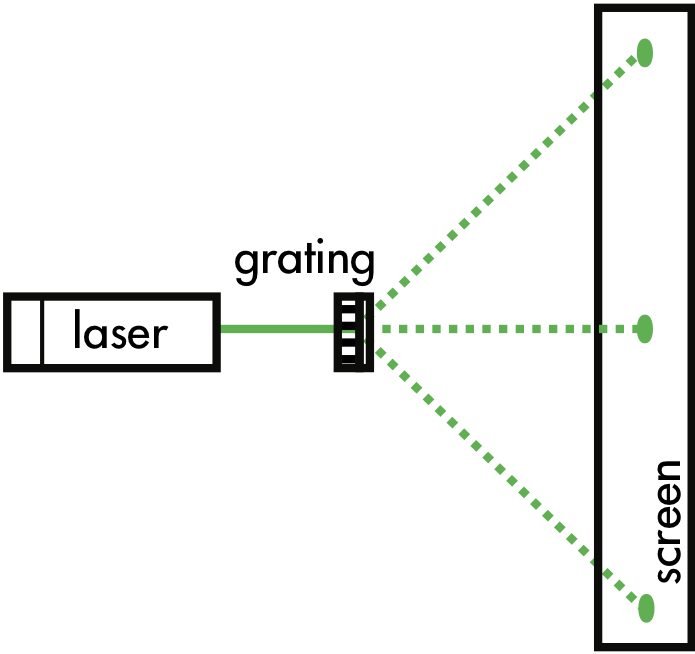## 20180324

### Physics midterm question: second maxima angles not possible

Physics 205B Midterm 1, spring semester 2018
Cuesta College, San Luis Obispo, CAA green laser (wavelength 550 nm) illuminates a grating with a unknown spacing between adjacent slits, producing an interference pattern with a first maxima angles of ±32°. (Drawing is not to scale.) Discuss why it is not possible for second maxima angles to be produced by this grating. Explain your reasoning using the properties of source phases, path lengths, and interference.

• p:
Correct. Discusses/demonstrates that the second maxima angle is not possible, by:
1. solving for the spacing d between grating slits, given the first maxima angle and the wavelength λ of the laser; then
2. solving for the second maxima angle results in a domain error for the inverse sine function, and interprets this as meaning that the there is no defined second maxima angle.
(It is possible to use d = λ/sin(32°) from the first maxima equation and insert it into the second equation without explicitly numerically solving for d, such that θ = sin−1(2/sin(32°) for the second maxima angle results in a domain error.)
• r:
As (p), but argument indirectly, weakly, or only by definition supports the statement to be proven, or has minor inconsistencies or loopholes. Typically has math errors.
• t:
Nearly correct, but argument has conceptual errors, or is incomplete. Uses minima equation and/or compounded math errors.
• v:
Limited relevant discussion of supporting evidence of at least some merit, but in an inconsistent or unclear manner. Some garbled attempt at applying properties of source phases, path lengths, and interference.
• x:
Implementation/application of ideas, but credit given for effort rather than merit. No clear attempt at applying properties of source phases, path lengths, and interference.
• y:
Irrelevant discussion/effectively blank.
• z:
Blank.
Sections 30882, 30883
Exam code: midterm01cVdP
p: 29 students
r: 2 students
t: 3 students
v: 1 student
x: 0 students
y: 0 students
z: 0 students

A sample "p" response (from student 1929):Another sample "p" response (from student 3481):